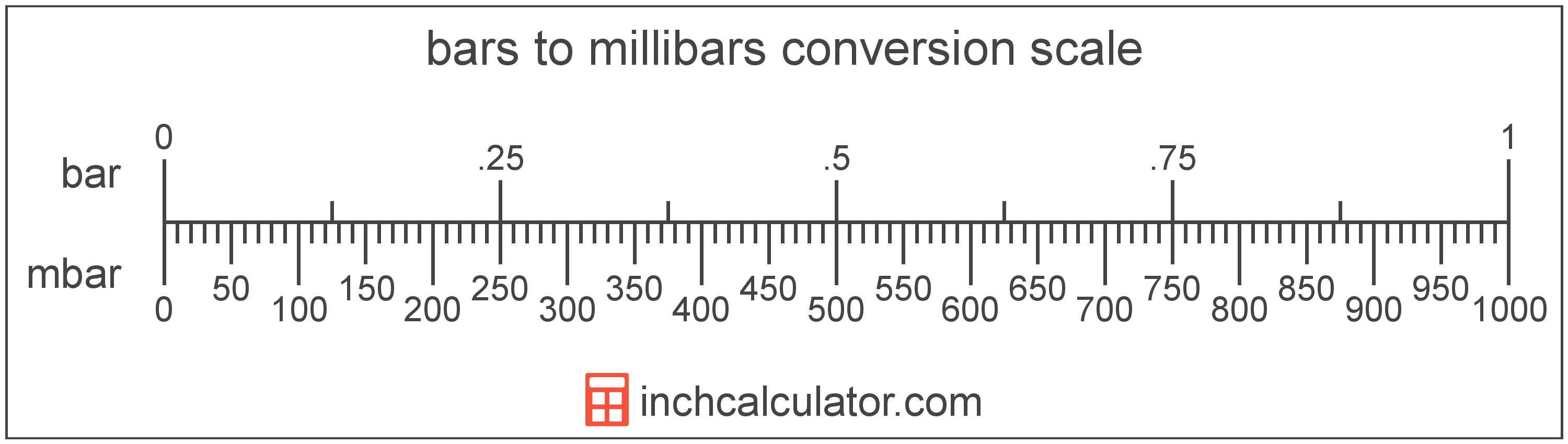# Bars to Millibars Converter

Enter the pressure in bars below to get the value converted to millibars.

Results in Millibars:1 bar = 1,000 mbar

Do you want to convert millibars to bars?

## How to Convert Bars to Millibars

To convert a measurement in bars to a measurement in millibars, multiply the pressure by the following conversion ratio: 1,000 millibars/bar.

Since one bar is equal to 1,000 millibars, you can use this simple formula to convert:

millibars = bars × 1,000

The pressure in millibars is equal to the pressure in bars multiplied by 1,000.

For example, here's how to convert 5 bars to millibars using the formula above.
millibars = (5 bar × 1,000) = 5,000 mbar## What is a Bar?

The bar is equal to 100,000 pascals, which are defined as the pressure of one newton per square meter. One bar is just less than the average atmospheric pressure.

The bar is a non-SI metric unit for pressure. Bars can be abbreviated as bar; for example, 1 bar can be written as 1 bar.

## What is a Millibar?

One millibar is equal to one-thousandth of a bar, which is defined as the pressure of one newton per square meter. One millibar is also equal to one hectopascal.

The millibar is a non-SI metric unit for pressure. In the metric system, "milli" is the prefix for thousandths, or 10-3. Millibars can be abbreviated as mbar; for example, 1 millibar can be written as 1 mbar.

## Bar to Millibar Conversion Table

Table showing various bar measurements converted to millibars.
Bars Millibars
0.001 bar 1 mbar
0.002 bar 2 mbar
0.003 bar 3 mbar
0.004 bar 4 mbar
0.005 bar 5 mbar
0.006 bar 6 mbar
0.007 bar 7 mbar
0.008 bar 8 mbar
0.009 bar 9 mbar
0.01 bar 10 mbar
0.02 bar 20 mbar
0.03 bar 30 mbar
0.04 bar 40 mbar
0.05 bar 50 mbar
0.06 bar 60 mbar
0.07 bar 70 mbar
0.08 bar 80 mbar
0.09 bar 90 mbar
0.1 bar 100 mbar
0.2 bar 200 mbar
0.3 bar 300 mbar
0.4 bar 400 mbar
0.5 bar 500 mbar
0.6 bar 600 mbar
0.7 bar 700 mbar
0.8 bar 800 mbar
0.9 bar 900 mbar
1 bar 1,000 mbar